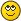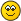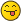Legendre's Duplication TheoremJmsNxnUltimate FellowPosts: 1,214 Threads: 126 Joined: Dec 2010 12/28/2022, 08:51 AM (This post was last modified: 12/28/2022, 09:26 AM by JmsNxn.) For those of you who don't know Legendre's Duplication Theorem; and for those you who do; I'm going to explain what it means in Fractional calculus (Where it relates fairly accuractely to Ramanujan's work). And it goes to further show the mysteries of the $\Gamma$ function, and how it is such a weird mysterious thing. I will then go on to talk about how it relates to our work here, when dealing with the Fractional Calculus approach of iteration theory. I want to go very slow for this post, as what I think I have my hands on is a Gaussian multiplication formula (which is just Legendre's Duplication to different orders). But to begin, let's define the $\Gamma$ function. We write: $\Gamma(s) = \int_0^\infty e^{-x} x^{s-1}\,dx\\$ This is the Eulerian integral expression for Gamma, but, Euler actually usually wrote it like: $s! = \int_0^1 (-\log(x))^{s}\,dx\\$ He later said that he preferred the integral: $s-1! = \int_0^\infty e^{-x} x^{s-1} \,dx$ Because of the singularity at $0$. Legendre took Euler at his word, and is solely responsible for the notation $\Gamma$, and the fact that $\Gamma(n) = (n-1)!$--so he takes all the heat. But Euler expressed that $(s-1)!$ seemed to have more importance than $s!$; hence the small change in variables. A quick proof of Legendre's Duplication Theorem is given at https://mathworld.wolfram.com/LegendreDu...rmula.html But it doesn't say much about what it means to us. Let's write: $\Gamma(2s) = \frac{2^{2s-1}}{\sqrt{\pi}} \Gamma(s) \Gamma(s+1/2)\\$ Now let's do mellin transforms; by first of all halving the variable $s$. $\int_0^\infty e^{-x} x^{s-1}\,ds = \frac{2^{s-1}}{\sqrt{\pi}} \Gamma(s/2) \Gamma((s+1)/2)\\$ But, we don't want to look at it like this, we want to actually take the inverse Mellin transform: $\frac{1}{2\pi i} \int_{1/2 - i\infty}^{1/2+ i \infty} \Gamma(s) x^{-s} \,ds = e^{-x}\\$ Whereby, if we change $\Gamma(s)$ to $\Gamma(2s)$, then: $\frac{1}{2\pi i} \int_{1/2 - i\infty}^{1/2+ i \infty} \Gamma(2s) x^{-s} \,ds = \frac{1}{\pi i} \int_{1/2 - i\infty}^{1/2+ i \infty} \Gamma(s) x^{-s/2} \,ds = 2e^{-\sqrt{x}}\\$ If we play around with these things, Legendre's Duplication theorem pops out pretty simply. We'll start with residues. When working with the Mellin transform; and when working with it in such a nice manner; all we care about are the poles to the left of the integral $\int_{1/2 - i \infty}^{1/2+i\infty}$. The first thing you should know about the $\Gamma$ function, is that it has poles at $k \le 0$, where $k$ is an integer. Additionally, $\Gamma(z+k) = \frac{(-1)^k}{|k|! z} + h(z)\\$ Where $h$ is holomorphic near $z = 0$. This gives the Mittag Leffler series (Again, proven by fucking Euler): $\Gamma(z) = \sum_{k=0}^\infty \frac{(-1)^k}{k!(z+k)} + \int_1^\infty e^{-x}x^{s-1}\,dx\\$ The essential deepness of Legendre's result is a little subtle, but it looks something like this.... The function $\Gamma(2s)$ has poles at $k$, where $k \in \mathbb{Z}/2$ and $k \le 0$. But, so does $\Gamma(s) \Gamma(s+1/2)$. They have poles at the exact same points. Now let's say, their Mittag Leffler expansion has the same residue; well to get that, all we need is $2^{2s-1}\Gamma(s)\Gamma(s+1/2)/\sqrt{\pi} = \Gamma(2s)$--where all we need is that this is the same at the poles. Their singular part is the same. We return to the integral now. If we brute force is; near every singularity: $\Gamma(2s) \sim \frac{2^{2s-1}}{\sqrt{\pi}}\Gamma(s) \Gamma(s+1/2)\\$ Now, let's prove Legendre's Duplication Theorem the long way. $\int_{1/2-i\infty}^{1/2+i\infty} \frac{2^{2s-1}}{\sqrt{\pi}}\Gamma(s) \Gamma(s+1/2) x^{-s} \,ds = 2 \pi i\sum_{k \in \mathbb{Z}/2,\,k\le 0} \text{Res}_{s= k} \frac{2^{2s-1}}{\sqrt{\pi}}\Gamma(s) \Gamma(s+1/2) x^{-s}\\$ We can make this statement, because both $\Gamma(2s)$ and $\Gamma(s)\Gamma(s+1/2)$ have the exact same decay at infinity in the complex plane... And more importantly, both integrals converge; and the Mellin transform is invertible (because it's just a fancy Fourier transform). Now let's, evaluate this integral with $x \mapsto x^2$, so I don't have to write a series with square roots. This entire thing just turns into.... $e^{-x}\\$ All we've done, is check that the principal part of Legendre's magic function, is the same as the principal part of $\Gamma(2s)$. But, additionally, Legendre's magic function, has enough decay to allow us to take this integral. Since this integral transform is $1-1$, and we can reduce the integral to it's residues--the result. This is not so much a novel proof of Legendre's Duplication Theorem--this is the route Gauss took to make the Gaussian Multiplication Theorem for The Gamma Function. So I'm basically quoting Gauss; I'm just focusing on the Fourier Transform language. There is nothing novel here, but it's nice to explain yourself. As to tetration; and iteration theory. I want to mimic this set up, but with iteration theory. For this, I'm going to take the familiar case of $f(z) = \sqrt{2}^{z+2}-2$--and I want to look at $f_- = f(-z)$ and the iteration of this. Obviously, we all know how to iterate $f$; but iterating $f_-$ can be tricky. To begin $\frac{1}{\sqrt{\pi}}\frac{\Gamma(s)\Gamma(1-s)}{\Gamma(\frac{s+1}{2})} (f_-\circ f_-)^{\circ -s} = h(s)$ is a holomorphic function. It is meromorphic in the left half plane, and has the appropriate decay at $s=\infty$ to be Mellin transformable. Where: $\frac{1}{2\pi i}\int_{1/2-i\infty}^{1/2 + i \infty} h(s)x^{-s}\,ds = \sum_{n=0}^\infty f_-^{\circ 2n} \frac{(-1)^nx^{2n}}{2n!} = \vartheta(x)\\$ We have to compare this to a different generating function; which we write as: $\vartheta_-(x) = \sum_{n=0}^\infty f_-^{\circ n} \frac{(-x)^n}{n!}\\$ Where by: $\frac{\vartheta_-(ix) +\vartheta_-(-ix)}{2} = \vartheta(x)\\$ In the same manner: $e^{ix} + e^{-ix} = 2\cos(\pi x/2)$...both of these functions are mellin transformable.... The function $\vartheta$ is transformable; and it looks like: $\int_0^\infty \vartheta(x)x^{s-1}\,dx = h(s)\\$ But; therefore: $\int_0^\infty \frac{\vartheta_-(ix) +\vartheta_-(-ix)}{2}x^{s-1}\,dx = h(s)\\$ The thing is; once you add distribution analysis, and advanced complex analysis. It doesn't matter how we take $\int_0^\infty$. Just that we start at the origin and eventually hit infinity; where then we rely on the decay of the integral. Thereby: $\int_0^\infty f(ix) x^{s-1}\,dx = i^{-s} \int_0^\infty f(x)x^{s-1}\,dx\\$ Where these are analytically continuable constructs. So long as we think of $\int_0^\infty$ as an integral on the Riemann Sphere; which means we go from $x=0$ to $x=\infty$ along some path. (It may be negative, it may be imaginary, it may be whatever the fuck). HUGE FUCKING EDIT RIGHT NOW; WE NEED SOME ROOT PI's HERE AND THERE. I AM JUST EXPLAINING THE GENERAL MOTIONS/PHILOSOPHY. I PROBABLY FORGOT SOME $\sqrt{\pi}$'s and some $2^s$'s or something stupid like that. I am just drawing out the picture. We actually get a Gaussian Multiplication Theorem https://mathworld.wolfram.com/GaussMulti...rmula.html But I'm not sure how to approach this yet.... Either way, we get The Legendre Duplication Theorem for Iteration theory: $f_-^{\circ -2s} \cos(\pi s) = h(s) = \frac{1}{\sqrt{\pi}}\frac{\Gamma(s)\Gamma(1-s)}{\Gamma(\frac{s+1}{2})} (f_-\circ f_-)^{\circ -s}$ My next goal is to fully rigorously derive this; and additionally derive Gauss's multiplication theorem. This gives a little tidbit though. We know that $f_-^{\circ \delta}(z) \to \infty$ if $\delta\to 0$. This is because there is no domain it is sending to. It hits the julia set no matter how you take it. So for that reason, when $s \to 0$ we tend to infinity no matter what. But it is balanced by the $\cos$ and the $\Gamma$ nonsense; such that it sticks to the same petal. But in order to stay in the petal it has to hit infinity. I believe I'm going to have to leave this forum permanently. And it's beccause I'm being reintroduced to deeper ideas. And the people reintroducing the ideas have a smarter purview.But Nonetheless, this effectively iterates $f(-z) = f_-(z)$ using the iterate of $f(z)$FOR FUCK SAKES. I'm missing some exponential shit, or what whatever. Fuck this shit. THIS IS ULTIMATELY JUST NEAR THE THE VALUE z=0 AND REQUIRES MORE FINESSE TO BE FULLY RIGOROUS « Next Oldest | Next Newest »

 Possibly Related Threads… Thread Author Replies Views Last Post Some "Theorem" on the generalized superfunction Leo.W 59 24,501 09/18/2022, 11:05 PM Last Post: tommy1729 tommy's new conjecture/theorem/idea (2022) ?? tommy1729 0 467 06/22/2022, 11:49 PM Last Post: tommy1729 tommy's singularity theorem and connection to kneser and gaussian method tommy1729 2 1,817 09/20/2021, 04:29 AM Last Post: JmsNxn  tommy's theorem sexp ' (z) =/= 0 ? tommy1729 1 6,097 06/17/2014, 01:25 PM Last Post: sheldonison Theorem on tetration. JmsNxn 0 3,416 06/09/2014, 02:47 PM Last Post: JmsNxn Vincent's theorem and sin(sexp) ? tommy1729 0 3,571 03/22/2014, 11:46 PM Last Post: tommy1729 number theory EPNT : extended prime number theorem tommy1729 0 3,801 08/23/2012, 02:44 PM Last Post: tommy1729 Carlson's theorem and tetration mike3 8 18,120 08/22/2010, 05:12 AM Last Post: bo198214 Weak theorem about an almost identical function: PROOF Kouznetsov 3 10,327 11/14/2008, 01:11 PM Last Post: Kouznetsov

Users browsing this thread: 1 Guest(s)